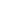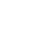×
Oil & Gas Training
and Competency Development1
• ;

#### Courses# Risk and Reliability Engineering

This course provides the basic knowledge and tools to determine the risk and reliability of equipment, a process and a system. It is designed to be 50% theoretical and 50% practical.

Day 1
• Introduction
• Basic Concepts
• Risk
• Risk Analysis
• Reliability
• Reliability Engineering
• Uncertainty – Uncertainty Management
• Asset Life Cycle Economic Analysis
• Deterministic Model
• Probabilistic Model
• Risk-Based Life Cycle Economic Analysis
• An Integrated Approach to Optimize the Economy of the Asset Life Cycle”
• Concept, Applications and Benefits
• Integrated Reliability Toolkit
• Disciplines
• Methodologies
Day 2
• Statistics for Risk and Reliability Analysis
• Basic Terms (Probability, Population, Sample, Descriptive Statistics, Random Variable)
• Descriptive Statistics
• Sample Statistics
• Histograms •
• Statistics (Sample Mean, Sample Mode, Sample Standard Deviation, Percentiles)
• Using Excel™ for sample statistics
• Population Statistics
• Probability Distribution Models
• Probability Distribution Formats (pdf, CDF, Inverse CDF)
• Statistics of probability distributions (Mean, Mode, Median,
• Probabilistic characterization of a sample or How to select a probability distribution model for a given sample.
• Goodness of fit Tests
• Use of software for goodness of fit tests. Statistics for Risk and Reliability Analysis
• Solving mathematical models (equations) random variable input parameters
• Sampling from a random variable
• Monte Carlo Simulation
• Using Excel™ for Monte Carlo Simulation
• Probabilistic Dependence
• Correlated Random Variables – Correlation Factor
• How to consider probabilistic dependence in Monte Carlo Simulation
Day 3
• Principles of Production Processes Reliability Analyisis
• Reliability, Availability and Maintainability (RAM)
• Analysis for Repairable Items
• Samples Data for RAM Analysis of repairable items
• Up-Time Data (Failure Data and Censored Data)
• Down Time Data
• Probability Distribution for Up -Time Samples in Repairable Items
• Probability Distribution for Down-Time Samples in Repairable Items
• Methodology for Availability and Expected Number of Failures calculations for Repairable items
• Availability and Expected Number of Failures Calculations
• Sources of Data for Reliability Analysis
• Evidence, Expert Opinion, Generic Data • Combining different sources of data
• Bayes Theorem
• Combining Generic Data with Evidence
• Combining Expert Opinion with Evidence
• System Availability and Expected Number of Failures Calculations
• Forecasting failures and availability of a pumping system
• Forecasting failures and availability in an ESP Oil Well
Day 4
• Principles of Financial Analysis
• Basics Terms and Definitions
• Life time of an investment , Projected Cash Flow, Discount Rate
• Financial Indicators • Net Present Value (Deterministic Model, Probabilistic Model)
• Annualized Present Value (Deterministic Model, Probabilistic Model)
• Principles of Risk Analysis
• Basic Terms and Definition
• Undesired Events
• Basic Model
• Qualitative and Semi-Quantitative Methods for Risk Analysis
• Quantitative Risk Analysis (QRA)
• An ESP Oil Well QRA Example
Day 5
• Risk Based Asset Economic Life Cycle Analysis
• General Model
• Monte Carlo calculation of NPV
• Sensitivity Analysis of Probabilistic NPV
• Determining the Risk Mitigation Actions Portfolio
• Comparing Investments Options – Risk / Profitability Matrix
• Final Exercise: Life Cycle Economic Analysis of an ESP Oil Well

Production engineers, Surface facilities Engineers, Reliability Engineers and Maintenance Engineers

Upcoming Classes

## Set a training goal, and easily track your progress

Customize your own learning journey and track your progress when you start using a defined learning path.In just few simple steps, you can customize your own learning journey in the discipline of your interest based on your immediate, intermediate and transitional goals. Once done, you can save it in NExTpert, the digital learning ecosystem, and track your progress.